# numpy.bitwise_or¶

numpy.bitwise_or(x1, x2[, out])

Compute bit-wise OR of two arrays, element-wise.

When calculating the bit-wise OR between two elements, x and y, each element is first converted to its binary representation (which works just like the decimal system, only now we’re using 2 instead of 10):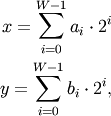where W is the bit-width of the type (i.e., 8 for a byte or uint8), and each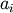and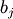is either 0 or 1. For example, 13 is represented as 00001101, which translates to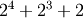.

The bit-wise operator is the result of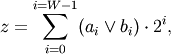where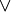is the OR operator, which yields one whenever eitheror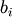is 1.

Parameters: x1, x2 : array_like Only integer types are handled (including booleans). out : array_like Result.

binary_repr
Return the binary representation of the input number as a string.

Examples

We’ve seen that 13 is represented by 00001101. Similary, 16 is represented by 00010000. The bit-wise OR of 13 and 16 is therefore 000111011, or 29:

>>> np.bitwise_or(13, 16)
29
>>> np.binary_repr(29)
'11101'

>>> np.bitwise_or(32, 2)
34
>>> np.bitwise_or([33, 4], 1)
array([33,  5])
>>> np.bitwise_or([33, 4], [1, 2])
array([33,  6])

>>> np.bitwise_or(np.array([2, 5, 255]), np.array([4, 4, 4]))
array([  6,   5, 255])
>>> np.bitwise_or(np.array([2, 5, 255, 2147483647L], dtype=np.int32),
...               np.array([4, 4, 4, 2147483647L], dtype=np.int32))
array([         6,          5,        255, 2147483647])
>>> np.bitwise_or([True, True], [False, True])
array([ True,  True], dtype=bool)


#### Previous topic

numpy.bitwise_and

#### Next topic

numpy.bitwise_xor